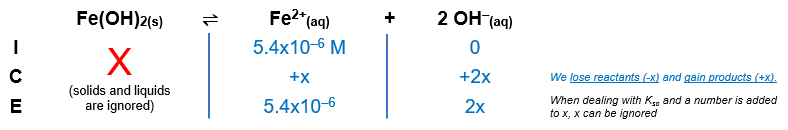# Problem: Iron concentrations greater than 5.4 × 10–6 M in water used for laundry purposes can cause staining. What [OH–] is required to reduce [Fe2+] to this level by precipitation of Fe(OH)2?

###### FREE Expert Solution

Fe(OH)2(s)  Fe2+(aq) + 2 OH(aq)The Ksp expression for Fe(OH)2 is:

${\mathbf{K}}_{\mathbf{sp}}\mathbf{=}\mathbf{\left[}{\mathbf{Ba}}^{\mathbf{2}\mathbf{+}}\mathbf{\right]}\mathbf{\left[}{{\mathbf{SO}}_{\mathbf{4}}}^{\mathbf{2}\mathbf{-}}\mathbf{\right]}\phantom{\rule{0ex}{0ex}}\mathbf{8}\mathbf{.}\mathbf{0}\mathbf{×}{\mathbf{10}}^{\mathbf{-}\mathbf{16}}\mathbf{=}\mathbf{\left(}\mathbf{5}\mathbf{.}\mathbf{4}\mathbf{×}{\mathbf{10}}^{\mathbf{-}\mathbf{6}}\mathbf{\right)}{\mathbf{\left(}\mathbf{2}\mathbf{x}\mathbf{\right)}}^{\mathbf{2}}\phantom{\rule{0ex}{0ex}}\mathbf{8}\mathbf{.}\mathbf{0}\mathbf{×}{\mathbf{10}}^{\mathbf{-}\mathbf{16}}\mathbf{=}\mathbf{\left(}\mathbf{5}\mathbf{.}\mathbf{4}\mathbf{×}{\mathbf{10}}^{\mathbf{-}\mathbf{6}}\mathbf{\right)}\mathbf{4}{\mathbit{x}}^{\mathbf{2}}\phantom{\rule{0ex}{0ex}}\frac{\mathbf{8}\mathbf{.}\mathbf{0}\mathbf{×}{\mathbf{10}}^{\mathbf{-}\mathbf{16}}}{\mathbf{2}\mathbf{.}\mathbf{16}\mathbf{×}{\mathbf{10}}^{\mathbf{-}\mathbf{5}}}\mathbf{=}\frac{\overline{)\mathbf{2}\mathbf{.}\mathbf{16}\mathbf{×}{\mathbf{10}}^{\mathbf{-}\mathbf{5}}}{\mathbf{x}}^{\mathbf{2}}}{\overline{)\mathbf{2}\mathbf{.}\mathbf{16}\mathbf{×}{\mathbf{10}}^{\mathbf{-}\mathbf{5}}}}\phantom{\rule{0ex}{0ex}}$

89% (293 ratings)###### Problem Details

Iron concentrations greater than 5.4 × 10–6 M in water used for laundry purposes can cause staining. What [OH] is required to reduce [Fe2+] to this level by precipitation of Fe(OH)2?

Frequently Asked Questions

What scientific concept do you need to know in order to solve this problem?

Our tutors have indicated that to solve this problem you will need to apply the Selective Precipitation concept. If you need more Selective Precipitation practice, you can also practice Selective Precipitation practice problems.

What professor is this problem relevant for?

Based on our data, we think this problem is relevant for Professor Dumont's class at McMaster University.

What textbook is this problem found in?

Our data indicates that this problem or a close variation was asked in Chemistry - OpenStax 2015th Edition. You can also practice Chemistry - OpenStax 2015th Edition practice problems.